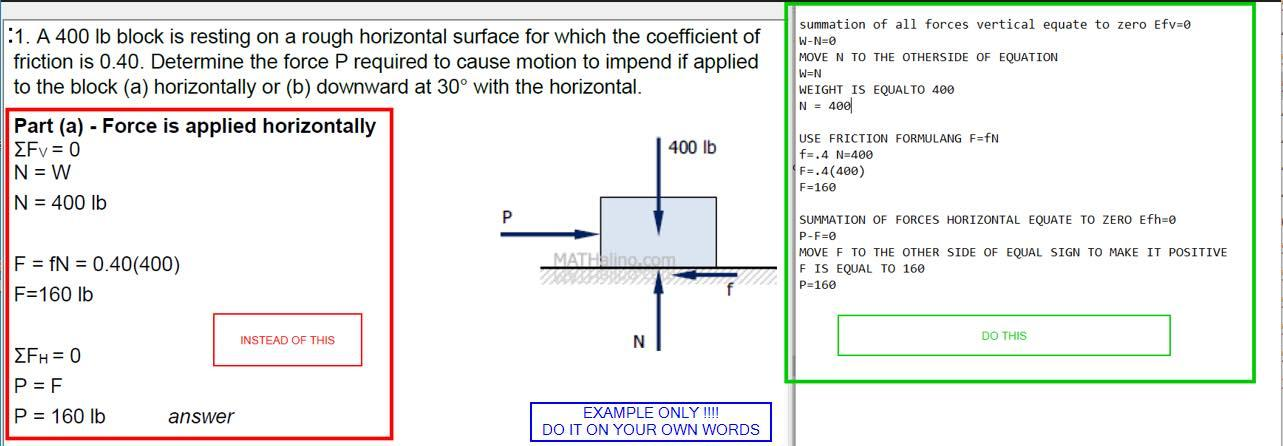Home / Expert Answers / Mechanical Engineering / a-400-lb-block-is-resting-on-a-rough-horizontal-surface-for-the-coefficient-of-friction-is-o-40-det-pa174

# (Solved): A 400 lb block is resting on a rough horizontal surface for the coefficient of friction is o.40. det ...

A 400 lb block is resting on a rough horizontal surface for the coefficient of friction is o.40. determine the force P required to cause motion to impend if applied to the block (A) horizontally or (B) downward at 30 degree with the horizontal1. A $$400 \mathrm{lb}$$ block is resting on a rough horizontal surface for which the coefficient of summation of all forces vertical equate to zero $$E f v=0$$ friction is $$0.40$$. Determine the force $$P$$ required to cause motion to impend if applied to the block (a) horizontally or (b) downward at $$30^{\circ}$$ with the horizontal. MOVE $$N$$ TO THE OTHERSIDE OF EQUATION $$\mathrm{W}=\mathrm{N}$$ WEIGHT IS EQUALTO 400 $$N=400 \mid$$ USE FRICTION FORMULANG $$F=f N$$ $$\mathrm{f}=.4 \mathrm{~N}=400$$ $$F=.4(400)$$ $$F=160$$ SUMMATION OF FORCES HORIZONTAL EQUATE TO ZERO EFh=0 $$\mathrm{P}-\mathrm{F}=0$$ MOVE F TO THE OTHER SIDE OF EQUAL SIGN TO MAKE IT POSITIVE F IS EQUAL TO 160 $P=160$

We have an Answer from Expert